/circle/slope-angles.html

Adapted from Geometry Labs, by Henri Picciotto

# Slope Angles

The ten-centimeter circle includes ruler and protractor markings.

1. Label the rulers in 1 cm increments.

2. Label the protractor in 15° increments. Start at 0° on the posititive x-axis, going counter-clockwise. Include angles greater than 180°.

3. Repeat Problem 2, going clockwise. In this direction, the angles are considered negative, so this time around, 345° will be labeled -15°.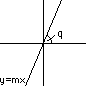Think of the line with equation y = mx, and of the angle q it makes with the positive x-axis. Each slope m corresponds to a certain angle. You can think about this relationship by drawing a right triangle on your 10-cm circle like one of those shown below. The legs give the rise and run for the slope of the hypotenuse. You can read off the angle where the hypotenuse crosses the protractor to find the angle that corresponds to a given slope. Note that even though both the examples below show positive slopes, you can use the circle geoboard to find the angles corresponding to negative slopes as well.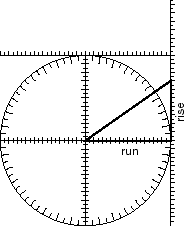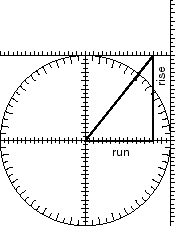1. The relationship between slope and angle is useful for solving practical problems, as you will see in later labs. But first, fill out the table below. Continue a pattern of going around the the circle to supply slopes where the table is blank.
m q
0
.2
.4
.6
.8
1
1.25
1.67

90°
-5

In Problem 4, you found angles that correspond to given slopes. You can also find slopes that correspond to given angles. Two examples of how to do this are shown below. In the example below left, you can read rise off the y-axis and run off the x-axis. In the example below right, a line is drawned at 30°, past the right edge. You can read rise off the right edge ruler. (What would run be in that example?)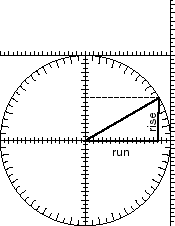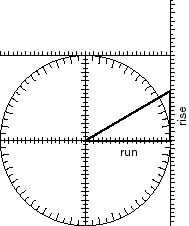1. Fill out the table below.
q m

15°
30°
45°
60°
75°
90°
105°
120°
135°
150°
165°
180°

## Discussion

1. What patterns do you notice when filling out the tables? What is the relationship between the slopes of complementary angles? For what angles is the slope positive, negative, 0? For what angles is the slope between 0 and 1? Greater than 1?

2. Why is there no slope for the angle of 90°?

3. Explain how you choose one or another of the types of slope triangles to help you fill out the tables.

4. Some of the slope triangles you used to fill out the tables are "famous right triangles". Check that the angles and slopes you found for those are correct.